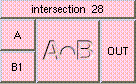INTERSECTIONTHE BOX'S MAIN HELP: The result is A * B. This is the intersection of A and B. A and B can be floats, points or colors, but they must be the same data type. Output will be the same data type. A (input): This may be a float, point, or color, but it must be the same type as B. The default is float. B (vinput): This may be a float, point, or color, but it must be the same type as A. The default is float. OUT (output): The result is A * B. This is the intersection of A and B. A and B can be floats, points or colors, but they must be the same data type. Output will be the same data type.

<-- BACK TO Math